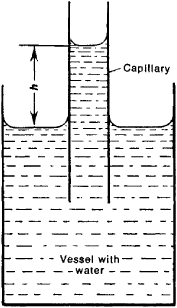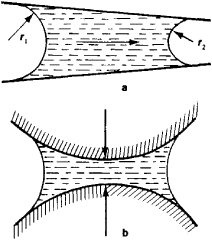Capillary Phenomena

The following article is from The Great Soviet Encyclopedia (1979). It might be outdated or ideologically biased.

Capillary Phenomena

physical phenomena caused by the surface tension at the interface of immiscible media. Capillary phenomena usually include those phenomena in liquid media caused by the curvature of their surface at their boundary with another liquid or gas or with their own vapors.

Surface curvature leads to the appearance of the additional capillary pressure A/? in the liquid, the magnitude of Δp being related to the average curvature r of the surface by Laplace’s equation, or Δp = p1p2 = 2σ/r, where σ12 is the surface tension at the boundary between the media and p1 and p2 are the pressures in liquid 1 and in medium (phase) 2 in contact with the liquid. In the case of a concave liquid surface (r < 0), the pressure in the liquid is lowered with respect to the neighboring phase: p1 <p2 and Δp Δ 0. The sign of Δp changes to the opposite sign for convex (r > 0) surfaces.

Capillary pressure is created by forces of surface tension acting in the direction of the tangent to the interface. Curvature of the interface leads to the appearance of a component directed into the volume of one of the contacting phases. For a flat interface (r = ∞) this component is nonexistent and Δp = 0.

Capillary phenomena include various cases of equilibrium and motion of the liquid surface under the action of both inter-molecular forces and external forces (mainly gravity).

In the simplest case, when external forces are either absent or compensated, the liquid surface is always curved. Thus, under the conditions of weightlessness, a limited volume of liquid that is not in contact with other bodies assumes the form of a sphere under the influence of surface tension. This form corresponds to the stable equilibrium of the liquid, since the sphere has the minimum surface for the given volume, and the surface energy of the liquid is therefore also minimal.

The liquid assumes the form of a sphere even if it is surrounded by another liquid of equal density (the effect of gravity is compensated by Archimedes’ principle, the force of buoyancy). The picture changes substantially when the force of gravity remains uncompensated. A low-viscosity liquid (for example, water) assumes the form of the vessel into which it is poured, provided that a sufficient amount of the liquid is used. The free surface of the liquid turns out to be practically flat, since the forces of gravity overcome the effect of surface tension, which tends to produce curvature and reduce the liquid surface. With decreasing liquid mass, however, the role of surface tension again becomes a determining factor: dispersion of a liquid in a gaseous medium or of a gas in liquid leads to the formation of small drops or bubbles that are practically spherical in shape.

The properties of systems consisting of many small droplets or bubbles (emulsions, liquid aerosols, and foams) and the conditions of their formation are to a large extent determined by the surface curvature of particles, that is, by capillary phenomena. Capillary phenomena are of equal importance in the formation of a new phase—of liquid droplets in steam condensation, of vapor bubbles in boiling liquids, and of solid-phase nuclei in crystallization.

The form of the contact surface between liquids and solids is substantially influenced by wetting phenomena, which are caused by molecular interactions between liquid and solid. Figure 1 shows a profile of the surface of a liquid wetting the wall of a vessel (see Figure 1). Wetting implies that a liquid interacts more strongly with the surface of a solid than does a gas over the liquid. The forces of attraction acting between the molecules of the solid and the liquid cause the latter to climb along the wall of the vessel, which leads to curvature of the surface region adjacent to the wall. This creates negative (capillary) pressure, which at each point of the curved surface precisely balances the pressure generated by the rise of the level of the liquid. The hydrostatic pressure within the volume of liquid remains unchanged in this case.Figure 1. Capillary rise of a wetting liquid (water in a glass vessel and capillary)

If the flat walls of the vessel are moved toward one another in such a way as to lead to superposition of the curved areas, a concave meniscus (a fully curved surface) is formed. The capillary pressure in a liquid is negative under the meniscus and causes the liquid to be sucked up into the slit to the point where the weight of the column of liquid (of height h) balances the active capillary pressure A/?. At equilibrium,

(ρ1 − ρ2)gh = Δp = 2σ12/r

where ρ 1 and ρ 2 are the densities of the liquid 1 and gas 2, respectively, and g is gravitational acceleration. This expression, known as Jurin’s equation (J. Jurin, 1684–1750), determines the height h of the capillary rise of a liquid that completely wets the walls of a capillary. A liquid that does not wet the surface forms a convex meniscus, which results in its drop in the capillary below the free surface level of the liquid (h < 0).

The capillary imbibition of liquids by porous bodies plays an essential role in supplying water to plants and in the propagation of moisture in soils and other porous bodies. Capillary impregnation of various materials is widely used in chemical engineering.

Curvature of the free surface of a liquid under the influence of external forces accounts for the existence of capillary waves (ripples on the surface of a liquid). The field of physicochemical hydrodynamics is concerned with the capillary phenomena observed during the movement of liquid interfaces.

The movement of a liquid in capillaries may be caused by differences in capillary pressure arising from differences in curvature of the liquid surface. The flow of liquid is in the direction of lower pressure. For wetting fluids the flow is in the direction of the meniscus with the shorter radius of curvature (Figure 2, a).Figure 2. (a) Movement of a liquid in a capillary through the influence of differences in capillary pressures (r, > r2), (b) contracting effect of capillary pressure

The vapor pressure (reduced according to Kelvin’s equation) over the wetting menisci is the cause of capillary condensation (of liquids) in fine pores.

Negative capillary pressure pulls together the walls that are restraining the liquid (Figure 2, b). This may lead to the considerable volume deformation of highly disperse systems and porous bodies, or capillary contraction. Thus, for example, the rise in capillary pressure that takes place during drying leads to considerable shrinkage in materials.

Many of the properties of disperse systems (permeability, strength, sorption of liquids) are determined to a large extent by capillary phenomena, since high capillary pressures are generated in the fine pores of these materials.

Capillary phenomena were discovered and studied by Leonardo da Vinci (15th century), B. Pascal (17th century), and J. Jurin (18th century) in experiments with capillary tubes. The theory of capillary phenomena was developed in the works of T. Young (1805), P. Laplace (1806), S. Poisson (1831), J. Gibbs (1875), and I. S. Gromeka (1879, 1886).

REFERENCES

Adam, N. K. Fizika i khimiia poverkhnostei. Moscow, 1947. (Translated from English.)
Gromeka, I. S. Sobranie sochinenii. Moscow, 1952.

N. V. CHURAEV